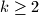### IMO Shortlist 1994 problem N3

Kvaliteta:
Avg: 0,0
Težina:
Avg: 7,0
Show that there exists a set$A$ of positive integers with the following property: for any infinite set$S$ of primes, there exist two positive integers$m$ in$A$ and$n$ not in$A$, each of which is a product of$k$ distinct elements of$S$ for some$k \geq 2$.
Izvor: Međunarodna matematička olimpijada, shortlist 1994